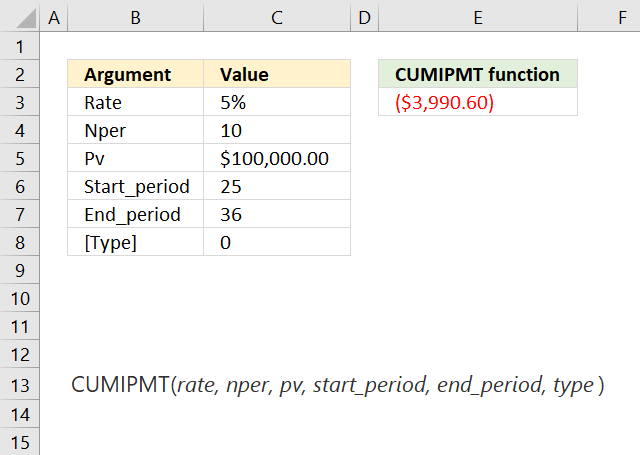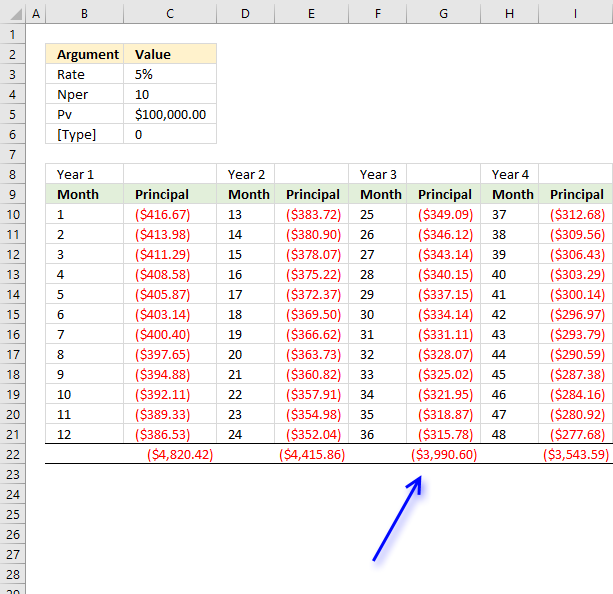Author: Oscar Cronquist Article last updated on May 11, 2022The CUMIPMT function calculates the accumulated interest based on a start and end period on a loan.

The image above shows the CUMIPMT function in cell E3 calculating the accumulated interest for month 25 to 36 for a 10 year loan of 100 000.

Formula in cell E3:

=CUMIPMT(C3/12,C4*12,C5,C6,C7,C8)

The first argument is the interest rate per year, we calculate the interest on a monthly basis so the argument has to be divide with 12, there are 12 months in a year.

The second argument is the number of periods the interest is calculated for, 10 year * 12 = 120 months or periods.

### Excel Function Syntax

CUMIPMT(rate, nper, pv, start_period, end_period, type)

### Arguments

 rate Required.  The interest rate. nper Required.  The total number of periods in an annuity. Examples: A monthly payment - 12 Quarterly payment - 4 pv Required.  Present value. start_period Required.  The first period. end_period Required. The last period. type Required.  When payments are due. 0 - End of period. 1 - At the beginning of the period.

The CUMIPMT function returns

• #NUM error value if:
• rate <= 0
• nper <= 0
• pv <= 0
• start_period < 1
• end_period < 1
• start_period > end_period
• type is not 0 (zero) or 1

Use the same unit for rate and nper, the above example uses monthly payments. That is why the interest rate is divided by 12 and nper is multiplied by 12. There are 120 monthly payments in a 10 year period.This image shows the interest for month 25 to 36 and the cumulative interest. The amount in cell G22 is the same as in the top image cell E3.